# Methods and formulas for estimation methods in Nonparametric Distribution Analysis (Right Censoring)

## Kaplan-Meier estimation

The Kaplan-Meier estimator, also known as the product limit estimator, can be used to calculate survival probabilities for nonparametric data sets with multiple failures and suspensions. The equation of the estimator is given by:

with S(t0) = 1 and t0 = 0.

### Empirical hazard function

The hazard function describes the rate of failure for an interval. The hazard function is 0 before the first censored observation. The hazard function changes only at uncensored observations. Minitab does not plot the hazard function after the last uncensored data point.

When there are ties, Minitab uses the largest rank in the tie to estimate the hazard function. See Nelson1 for more details.

### Mean time to failure

For uncensored data, the mean time to failure is the same as the average failure time. The general formula to use with censored or uncensored data follows:

Also, when the largest observation is censored, Minitab treats the time of the largest uncensored observation as a time limit for the calculation. See Lee2 for more details.

### Standard error of MTTF

The standard error of the mean time to failure is the square root of the variance. When all observations are uncensored, Minitab calculates an unbiased estimate:

For the cases where some data are censored, the unbiased estimate of the variance is the following formula:

Because of the shape of the empirical hazard function, the areas under the survival curve, Ar, are rectangles with heights equal to the survival function and lengths equal to the intervals between uncensored observations.

### Notation

TermDescription
tr time of the data point with rank r
rrank of the data point, where the earliest failure has the lowest rank
ntotal number of units
δr 0 if the jth observation is censored or 1 if the jth observation is uncensored
cnumber of data points until the next uncensored observation
S(tr)empirical survival function at time tr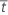average failure stress
Ararea under the curve of the survival plot to the right of tr
mtotal number of uncensored observations

### References

1. W. Nelson (1982). Applied Life Data Analysis. John Wiley & Sons, Inc. 133.

2. Elisa T. Lee (1992). Statistical Methods for Survival Data Analysis, Second Edition. John Wiley & Sons, Inc. 73-76.

## Actuarial estimation

The actuarial model is an alternative nonparametric analysis that displays information for groupings of failure times. The Kaplan-Meier method assumes that the suspensions in an interval occur at the end of that interval, after the failures have occurred. Minitab's actuarial model assumes that the suspensions occur in the middle of the interval, which has the effect of reducing the number of available units in the interval. The estimate of the survival function using the actuarial method is as follows: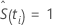for i = 0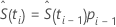for i > 0

### Empirical hazard function

The hazard function describes the rate of failure for an interval. With actuarial estimation, you assume that the calculation is for the midpoint of the interval. On the hazard plot, the function is drawn from midpoint to midpoint. For more details, see the references following the Notation section.

### Notation

TermDescription
ni the number of units entering an interval
di the number failing in the interval
n'i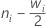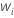the number censored in an interval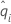the conditional probability of an event, which equals di/n'i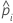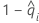tmitime at the midpoint of the actuarial interval
bithe length of the actuarial interval

### References

Collett, D. (1994) Modelling Survival Data in Medical Research, Chapman and Hall.

Lee, Elisa T. (1992) Statistical Methods for Survival Data Analysis, 2nd Edition, John Wiley & Sons.

## Turnbull estimation

Turnbull1, 2 developed an iterative algorithm to compute a nonparametric maximum likelihood estimate of the cumulative distribution function for the data. This method applies to more general situations, for example, where the intervals are overlapping.

Minitab displays output summarizing the Turnbull estimate of the interval probabilities, along with standard errors for these probabilities.

### References

1. B.W. Turnbull (1976). "The Empirical Distribution Function with Arbitrarily Grouped, Censored and Truncated Data," Journal of the Royal Statistical Society 38, pp. 290-295.
2. B.W. Turnbull (1974). "Nonparametric Estimation of a Survivorship Function with Doubly Censored Data," Journal of the American Statistical Association 69, 345, pp. 169-173.

## Confidence intervals

Regardless of the estimation method, Minitab uses a normal approximation to calculate confidence intervals. The confidence intervals are as follows:

### Formula

Estimate of the survival probability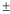zα × Standard error of the estimate

### Notation

TermDescription
zα the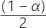upper critical value for the standard normal distribution
α the confidence level
By using this site you agree to the use of cookies for analytics and personalized content.  Read our policy